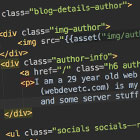## JavaScript: How to convert between types in JS?

If you have some variable in JS, you might end up needing to convert it to another data type. For example, to change from a string to an integer value. Here is how to do it.

## Converting to a string

### Convert From number to string in JS

If you wrap a number in `String( some_int_or_float )`, it will return a string object.

`let numeric_val = 1234;console.log(numeric_val);console.log(typeof numeric_val); let string_from_numeric = String( numeric_val) ;console.log(string_from_numeric);console.log(typeof string_from_numeric);`
```1234
number
1234
string```

Alternatively you can just do `.toString()` on a number variable and it will return a string.

`let num = 123;let num_as_string = num.toString();`

### Convert from a boolean to a string in JS

To convert from a boolean to a string, it follows a similar pattern as from a number to a string. Just wrap it in `String ( true ) ` and it will return a 4 char string 'true' (or 'false' ).

`let your_bool = false;console.log(false);console.log(typeof false); let string_from_your_bool = String( your_bool) ;console.log(string_from_your_bool);console.log(typeof string_from_your_bool);`
```false
boolean
false
string```

You can also use the `.toString()` method:

`let your_bool = false;your_bool_as_string_2 = your_bool.toString();console.log("Type: " + (typeof your_bool_as_string_2) + " and its value: " + your_bool_as_string_2);`
`Type: string and its value: false`

### How to convert from an array to a string in JS

To convert from an array to a string in Javascript, you just wrap an array in `String( ['a','b','c'] )` and it will return a comma separated list of the items.

`let array = [100,200,333,444];let array_as_string = String(array);console.log(array_as_string);// logs the string "100,200,333,444"`

### Convert from a date to a string in JS

You can wrap a Data object in `String ( new Date )` to get it as a string.

`let some_date = new Date;console.log(some_date);console.log(typeof some_date); let string_from_some_date = String( some_date ) ;console.log(string_from_some_date);console.log(typeof string_from_some_date);`
```Wed Jan 12 2016 01:50:10 GMT+0200 (Central European Summer Time)
object
Wed Jan 12 2016 01:50:10 GMT+0200 (Central European Summer Time)
string```

## How to convert to numbers

### How to convert from a string to a number:

To convert from a string (which contains a number) you can do it like this:

`let a_number_as_a_string = '1234'; // this is a number let converted_to_a_number = Number( a_number_as_a_string ); // now it is a number!// alternative ways to do this: // as an integerlet int_value = parseInt(a_number_as_a_string);// as a float:let float_value = parseFloat(a_number_as_a_string);let float_value_2 = parseFloat("3.14");`

However if you tried to convert a string such as `'asdfg'` it would return `NaN` (not a number) as obviously there is no way to convert 'asdfg' to a number.

### Convert a boolean to a number in JS

This will convert bools to numbers. A true value gets converted to 1, and false to 0. Null (which isn't a boolean) also would convert to 0.

`let a_boolean = true; let converted_to_a_number = Number( a_boolean ); // now it is a number! it's value is 1`

## Automatic type coercion

JS can do some conversion without you having to explicitly tell it to.

For example, in the following code because it starts with a string (`a_string`), when it gets to the `+ a_number` it will convert a_number to its string value.

`let a_string = "Hello, world ";let a_number = 1234; console.log(a_string + a_number);`
`Hello, world 1234`webdevetc

I am a 29 year old backend web developer from London, mostly focusing on PHP and Laravel lately. This (webdevetc.com) is my blog where I write about some web development topics (PHP, Laravel, Javascript, and some server stuff). contact me here.

### More...

##### Or see other languages/frameworks:
PHP Laravel Composer Apache CentOS and Linux Stuff WordPress General Webdev and Programming Stuff JavaScript